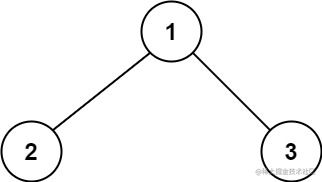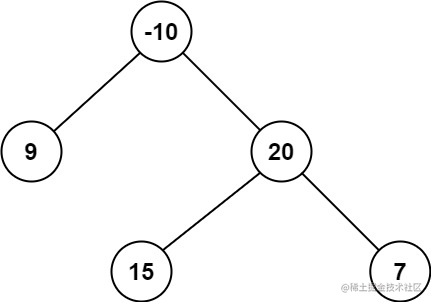# 前端算法必刷题系列

## 130. 最小栈 (min-stack)

• Stack
• 简单

### 题目

leetcode 传送门

push(x) —— 将元素 x 推入栈中。 pop() —— 删除栈顶的元素。 top() —— 获取栈顶元素。 getMin() —— 检索栈中的最小元素

pop、top 和 getMin 操作总是在 非空栈 上调用。

``````输入：
["MinStack","push","push","push","getMin","pop","top","getMin"]
[[],[-2],,[-3],[],[],[],[]]

[null,null,null,null,-3,null,0,-2]

MinStack minStack = new MinStack();
minStack.push(-2);
minStack.push(0);
minStack.push(-3);
minStack.getMin();   --> 返回 -3.
minStack.pop();
minStack.top();      --> 返回 0.
minStack.getMin();   --> 返回 -2.

### 写法实现

``````var MinStack = function() {
this.stack = []
this.minStack = []
};

/**
* @param {number} val
* @return {void}
*/
MinStack.prototype.push = function(val) {
this.stack.push(val)
if (this.minStack.length === 0 || val <= this.getMin()) {
this.minStack.push(val)
}
};

/**
* @return {void}
*/
MinStack.prototype.pop = function() {
// 判断出栈的是否是最小元素，是的话就把最小栈顶推出
if (this.getMin() === this.stack.pop()) {
this.minStack.pop();
}
};

/**
* @return {number}
*/
MinStack.prototype.top = function() {
return this.stack[this.stack.length - 1]
};

/**
* @return {number}
*/
MinStack.prototype.getMin = function() {
// 取最小栈的栈顶元素，就是最小的
return this.minStack[this.minStack.length - 1];
};

## 131. 二叉树中的最大路径和 (binary-tree-maximum-path-sum)

• 二叉树
• 困难

### 题目

leetcode 传送门``````输入：root = [1,2,3]``````输入：root = [-10,9,20,null,null,15,7]

### 基本思路

• 递归的核心就是找最小子问题，本题的核心就是考虑实现一个简化的函数 `maxGain(node)`，该函数计算二叉树中的一个节点的最大贡献值，具体而言，就是在`以该节点为根节点的子树中`寻找以该节点为起点的一条路径，使得该路径上的节点值之和最大

• 思考递归问题，不要纠结细节实现屏蔽细节地思考。递归就两要素，`1. 调用自身，2. 递归出口`

• 结点有可能是`负值`，递归发现左右节点贡献为负，需要舍弃，直接为 0，就是不往下走。

### 写法实现

``````var maxPathSum = function(root) {
let maxSum = Number.MIN_SAFE_INTEGER;

// 求最大路径的函数
const maxGain = (node) => {
// 递归到 null 直接返回 0，路径不增加
if (node === null) {
return 0
}
// 递归计算左右子节点的最大贡献值，
// 注意要大于 0 时再累计，否则直接为 0，因为节点值可能是负的

let leftGain = Math.max(maxGain(node.left), 0);
let rightGain = Math.max(maxGain(node.right), 0);

// 节点的最大路径和取决于该节点的值与该节点的左右子节点的最大贡献值
let newpath = leftGain + node.val + rightGain;

// 跟历史最大比较
maxSum = Math.max(maxSum, newpath);

// 返回节点的最大路径
return node.val + Math.max(leftGain, rightGain);
}

maxGain(root);
return maxSum;
};